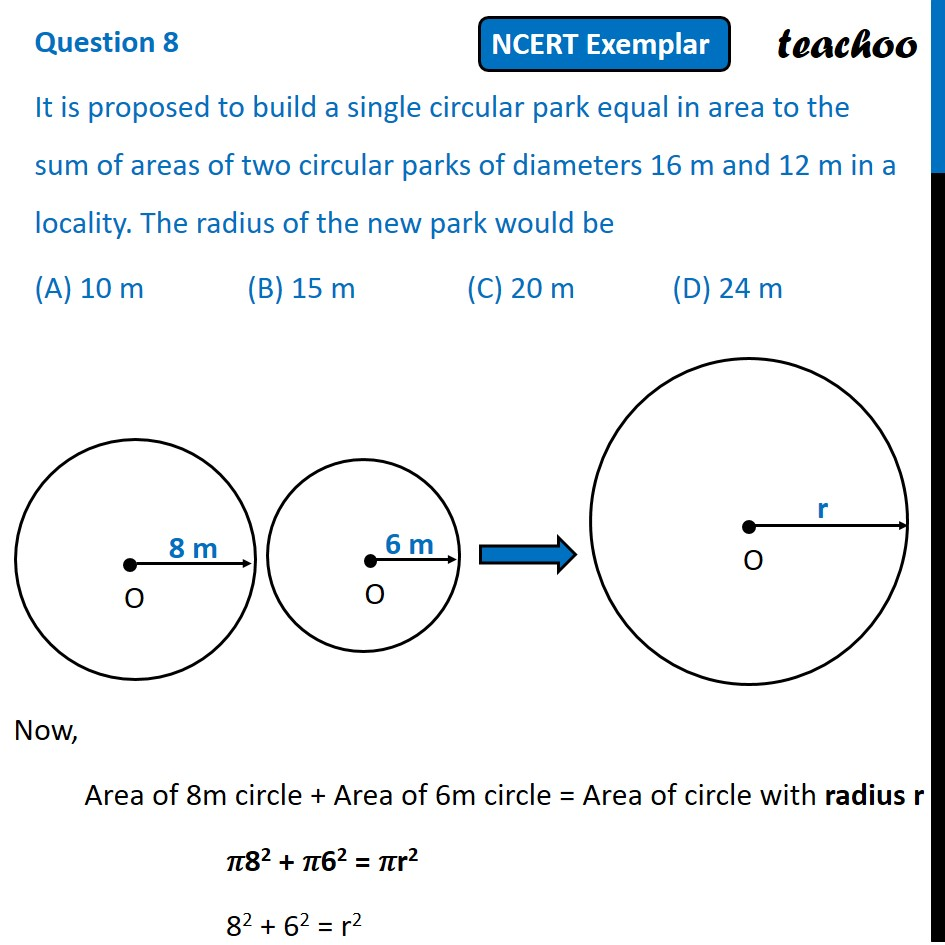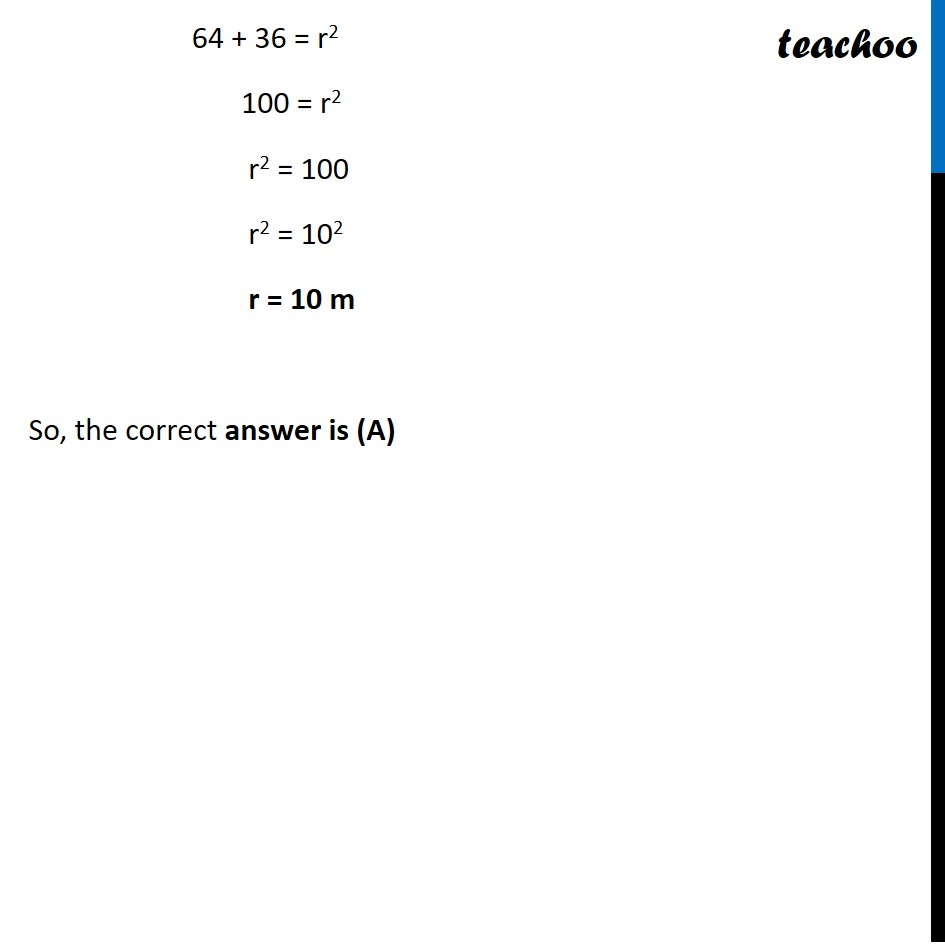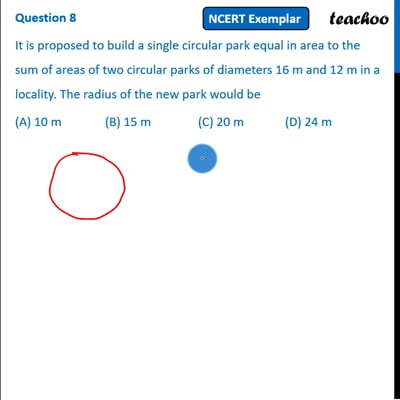NCERT Exemplar - MCQ

Chapter 11 Class 10 Areas related to Circles
Serial order wise

## (A) 10 m   (B) 15 m  (C) 20 m   (D) 24 mThis video is only available for Teachoo black users

Learn in your speed, with individual attention - Teachoo Maths 1-on-1 Class

### Transcript

Question 8 It is proposed to build a single circular park equal in area to the sum of areas of two circular parks of diameters 16 m and 12 m in a locality. The radius of the new park would be (A) 10 m (B) 15 m (C) 20 m (D) 24 m Now, Area of 8m circle + Area of 6m circle = Area of circle with radius r 𝜋82 + 𝜋62 = 𝜋r2 82 + 62 = r2 64 + 36 = r2 100 = r2 r2 = 100 r2 = 102 r = 10 m So, the correct answer is (A)